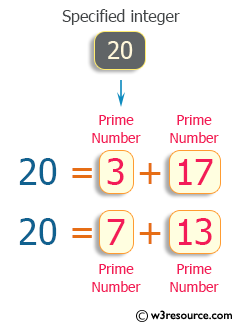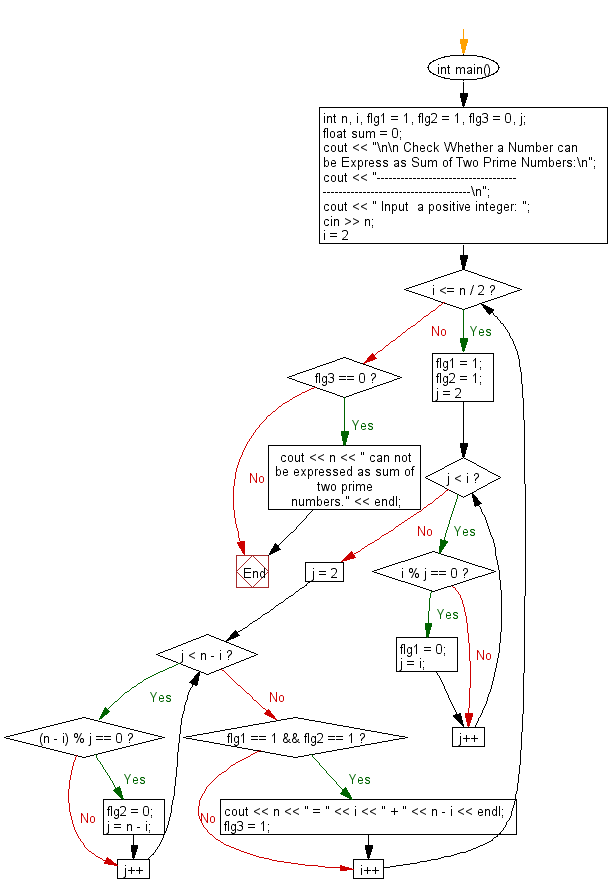﻿ C++ : Check if a number can be Express as Sum of two Primes

# C++ Exercises: Check Whether a Number can be Express as Sum of Two Prime Numbers

## C++ For Loop: Exercise-33 with Solution

Write a program in C++ to check whether a number can be expressed as the sum of two.

Visual Presentation:Sample Solution:-

C++ Code :

``````#include <iostream> // Include the input/output stream library
using namespace std; // Using standard namespace

int main() // Main function where the execution of the program starts
{
int n, i, flg1 = 1, flg2 = 1, flg3 = 0, j; // Declare integer variables n, i, flg1, flg2, flg3, j
float sum = 0; // Declare and initialize a float variable sum to 0

// Display message asking for input
cout << "\n\n Check Whether a Number can be Express as Sum of Two Prime Numbers:\n";
cout << "------------------------------------------------------------------------\n";
cout << " Input  a positive integer: ";
cin >> n; // Read input for a positive integer

// Loop to find two prime numbers whose sum equals the input number
for (i = 2; i <= n / 2; i++)
{
/*---------- check for prime---------------*/
flg1 = 1; // Initialize flg1 as 1 (considering the number is prime)
flg2 = 1; // Initialize flg2 as 1 (considering the number is prime)

// Loop to check if 'i' is a prime number
for (j = 2; j < i; j++)
{
if (i % j == 0) // If 'i' is divisible by any number other than 1 and itself
{
flg1 = 0; // Set flg1 as 0 (indicating 'i' is not prime)
j = i; // Exit the loop
}
}

// Loop to check if 'n - i' is a prime number
for (j = 2; j < n - i; j++)
{
if ((n - i) % j == 0) // If 'n - i' is divisible by any number other than 1 and itself
{
flg2 = 0; // Set flg2 as 0 (indicating 'n - i' is not prime)
j = n - i; // Exit the loop
}
}

// If both 'i' and 'n - i' are prime numbers, print their sum as 'n'
if (flg1 == 1 && flg2 == 1)
{
cout << n << " = " << i << " + " << n - i << endl; // Display the expression of 'n' as sum of two prime numbers
flg3 = 1; // Set flg3 as 1 to indicate a successful expression of 'n'
}
}

// If 'n' cannot be expressed as the sum of two prime numbers, display a message
if (flg3 == 0)
{
cout << n << " can not be expressed as sum of two prime numbers." << endl; // Display that 'n' cannot be expressed as the sum of two prime numbers
}
}
``````

Sample Output:

``` Check Whether a Number can be Express as Sum of Two Prime Numbers:
------------------------------------------------------------------------
Input  a positive integer: 20
20 = 3 + 17
20 = 7 + 13
```

Flowchart:C++ Code Editor: